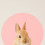# SBI PO Data Interpretation Online Practice Tests - Mixed Graphs

Dear SBI PO Online Exam 2014 aspirants, we have already discussed in detail about the concept of Data Interpretation, along with examples of various types. Today we shall discuss about the last type of Data Interpretation, which is Mixed Graphs. In mixed graphs, instead of giving the data in any of the standard formats, they merge two or three formats as one. You have to study them to answer the given questions. Below is a mixed graph which is a combination of bar chart and table. Before going to take the test, read the introductory lessons from below links. Happy Reading :)

The total number of students passed from six different schools is 8000. The given bar-graph shows the proportion of students who passed the exam and the table shows the percentage of students who got 1st, 2nd and 3rd class in the exam. Study the given data and answer the following questions.

 1st 2nd 3rd E1 23 45 32 E2 29 48 23 E3 35 30 35 E4 44 24 32 E5 42 36 22 E6 38 37 25

1. What is the difference between the total number of students who passed from School E1, E2 and E3 together and that of those who passed from School E4, E5 and E6 together ?
1. 360
2. 400
3. 420
4. 450
5. 480
Solution :
(E1 + E2 + E3) = ((8 + 5 + 6) / 40) x 8000

= 3800

(E4 + E5 + E6) = 4200

So, the difference is 400

i.e., Option 2

2. What is the total number of students who got 1st class in the exam from all the schools together ?
1. 2350
2. 2792
3. 2858
4. 2916
5. 2994
Answer : 2858 i.e., Option 3

3. What is the ratio of the number of students who got 2nd class from School E3 to the number of students who got 3rd class from School E6 ?
1. 8:3
2. 9:5
3. 7:4
4. 9:7
5. 5:2
Solution :
(E3)Second = 1200 x (30 / 100) = 360

(E6)Third = 800 x (25/100) = 200

So, the ratio = 360/200 = 9/5

i.e., Option 2

4. Total number of students who got in 3rd class in School E5, is what percentage of the total number of students passing from all schools together ?
1.  2.75%
2. 3.25%
3. 4.5%
4. 5.6%
5. 6.3%
Solution :
(E5)Third = 1000 x (22/100) = 220
So, required % = (220/8000) x 100 = 2.75 %
i.e., Option 1

5. The number of students who got 3rd class in school E1 is what percentage more than the number of students who got 1st class from School E3 ?
1. 17.8%
2. 21.9%
3. 24.6%
4. 27.2%
5. 28.6%
Solution :

(E1)Third = 1600 x (32/100) = 512

(E3) First = 1200 x (35/100) = 420

So, Required % = ((512-420) / 420) x 100 = 9200/420

This is approximately equal to 21.9%

i.e., Option 2

1.plz upload capsule for sbi po make it fast

2.Mam how you put 40 at bottom in first solution?
And
In
3rd one the 1200 and 800?

1.Same question here..pls explain us

2.Hi.
in 1st ques 40 comes from add all proportions (8+5+6+12+5+4).

in 2nd ques first find school total student eg
E1 : (8X 8000)/40=1600.
now total students passed with !st Class : 1600 X 23%=368.
similarity find out other school students and then add them and that is anwser.

in 3rd ques 1200 and 800 comes from proportion bar graph
E3 : (6X 8000)/40=1200
E6 : (4 X 8000)/40=800

3.4.40 is total of all bars

3.mam pls give us solution of 2nd question

1.assume 8000=40 then 1 part of 8000=200 question asked al 1st class sutdnts means
in bar graph e1=8parts so 8*200=1600 passed students in that 23% 1st class means 1600*23/100=368
same process to e2 e3 e4 e5 e6 and finally add all sums=2850

4.This comment has been removed by the author.

5.Samaj nai aah raha...koi samjao

1.Which part you are not understanding?

6.Mam plz keep on posting these type of data interpretation,

Thanks lot

7.mam can u plz tell what question otther than d.i. will be there in apt sec, sbi po plz mam

8.Plz explain it

9.Plz explain the 2nd question??

10.Its a very nice set od D.I., please post some more useful sets please

11.40 in the bottom is the sum of the proportion of all the schools ie E1+E2+E3+E4+E5 i.e (8+5+6+12+5+4)=40

12.hai please any one explain 2nd one?

13.pls explian 2nd one?

14.Plz expln 2nd

15.Thakyou well sone

16.8/40*8000=1600
E1=1600*23/100=368 similaraly we can calculate others